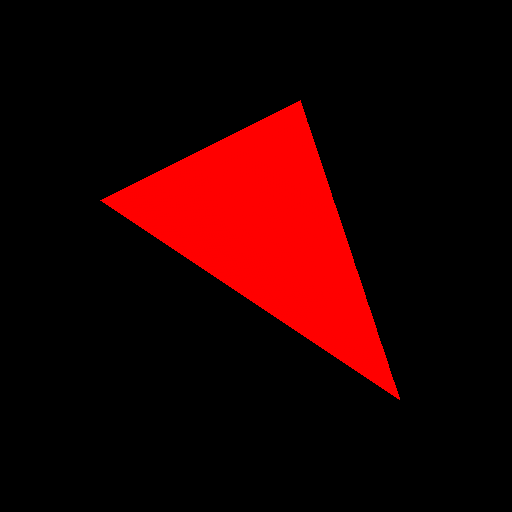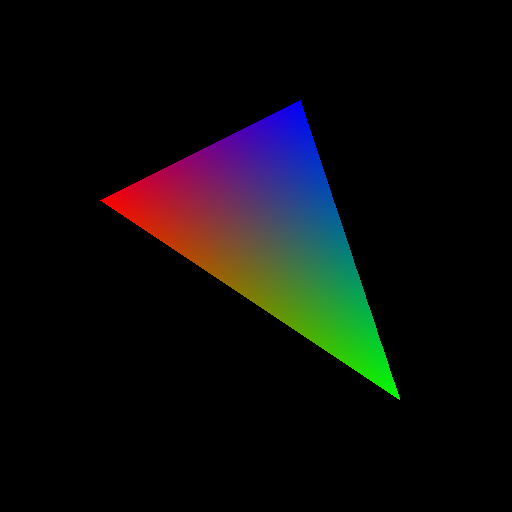# Lab 2 - Rasterizer: Single Triangle

## Overview and Context

We are continuing to build some basic tools for Assignment 1.

## Goals for This Lab

For this lab, you will add onto your first lab and only rasterize a single triangle. Your program must:

• Read in three vertices which represent a triangle.
• Read in different color values to each vertex.
• Compute the bounding box of the triangle using your Lab01 code.
• Compute the barycentric coordinates for every pixel in the bounding box.
• For any pixel that falls in the triangle, color that pixel with the interpolated color using the barycentric coordinates to weight the vertex colors.
• Write out those pixels as an image.

## Step 0

Copy over CMakeLists.txt and the src folder from the previous lab. Change the name of the project in the CMake file. Build and run the executable as before. E.g., with command line,

> mkdir build
> cd build
> cmake ..
> make -j4
> ./Lab02

With Xcode:

> mkdir build
> cd build
> cmake -G Xcode ..
> # Open Lab02.xcodeproj with Xcode 

With Visual Studio:

> mkdir build
> cd build
> cmake ..
> # Open Lab02.sln with Visual Studio

## Step 1

Starting from your Lab01 code, add command line arguments to read-in three different red, green, blue values to the three different vertices. (Consider differing colors that make a blended color you can reason about such as red and blue or yellow and blue – look up any RGB color chart to pick good colors.) The command line arguments should be:

• Output filename (e.g., foo.jpg)
• Image width (e.g., 512)
• Image height (e.g., 512)
• Vertex 1 x-coord (e.g., 100)
• Vertex 1 y-coord (e.g., 100)
• Vertex 1 red (e.g., 255)
• Vertex 1 green (e.g., 0)
• Vertex 1 blue (e.g., 255)
• Vertex 2 x-coord
• Vertex 2 y-coord
• Vertex 2 red
• Vertex 2 green
• Vertex 2 blue
• Vertex 3 x-coord
• Vertex 3 y-coord
• Vertex 3 red
• Vertex 3 green
• Vertex 3 blue

## Step 2

Using your prior code, compute the bounding box of the triangle. Now for every pixel in the bounding box, compute the barycentric coordinates for each pixel. You may “borrow” some code for this from the web, but if you do, make sure to cite the source in your code (and README for assignment).

Draw the triangle, rather than just the bounding box, by using the fact that any pixel that falls within the triangle will have $$\alpha$$, $$\beta$$, and $$\gamma$$ between 0.0 and 1.0. For debugging this step, ignore the colors from the previous step and try drawing a red triangle.## Step 3

For any pixel that will be drawn, its color should be a blend of the colors specified at the triangle vertices. Blend the colors using $$\alpha$$, $$\beta$$, and $$\gamma$$ that were computed in the barycentric coordinate computation. See the example figure below for an idea of what your rasterized triangle should look like.Generated on Thu Jan 9 11:48:50 CST 2020